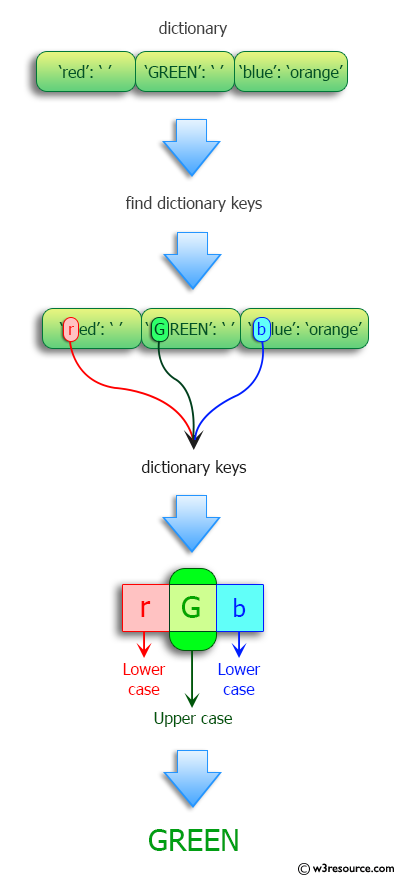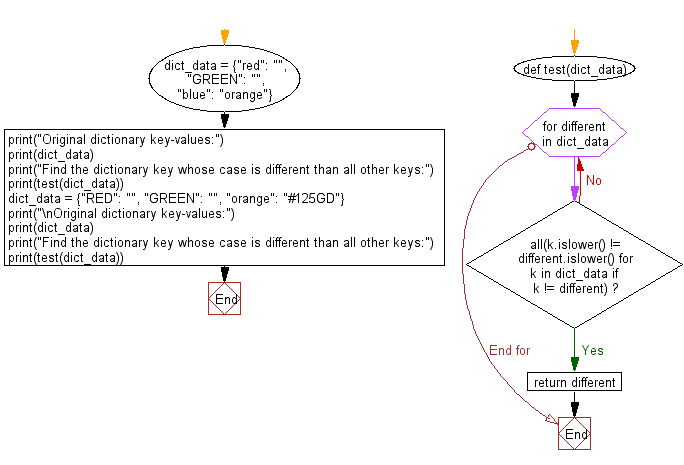﻿ Python: Find the dictionary key whose case is different than all other keys - w3resource# Python: Find the dictionary key whose case is different than all other keys

## Python Programming Puzzles: Exercise-62 with Solution

Write a Python program to find the dictionary key whose case is different than all other keys.

```Input:
{'red': '', 'GREEN': '', 'blue': 'orange'}
Output:
GREEN

Input:
{'RED': '', 'GREEN': '', 'orange': '#125GD'}
Output:
orange
```

Pictorial Presentation:Sample Solution:

Python Code:

``````#License: https://bit.ly/3oLErEI

def test(dict_data):
for different in dict_data:
if all(k.islower() != different.islower() for k in dict_data if k != different):
return different

dict_data = {"red": "", "GREEN": "", "blue": "orange"}
print("Original dictionary key-values:")
print(dict_data)
print("Find the dictionary key whose case is different than all other keys:")
print(test(dict_data))
dict_data = {"RED": "", "GREEN": "", "orange": "#125GD"}
print("\nOriginal dictionary key-values:")
print(dict_data)
print("Find the dictionary key whose case is different than all other keys:")
print(test(dict_data))
``````

Sample Output:

```Original dictionary key-values:
{'red': '', 'GREEN': '', 'blue': 'orange'}
Find the dictionary key whose case is different than all other keys:
GREEN

Original dictionary key-values:
{'RED': '', 'GREEN': '', 'orange': '#125GD'}
Find the dictionary key whose case is different than all other keys:
orange
```

Flowchart:## Visualize Python code execution:

The following tool visualize what the computer is doing step-by-step as it executes the said program:

Python Code Editor :

Have another way to solve this solution? Contribute your code (and comments) through Disqus.

What is the difficulty level of this exercise?

Test your Programming skills with w3resource's quiz.

﻿

## Python: Tips of the Day

Clamps num within the inclusive range specified by the boundary values x and y:

Example:

```def tips_clamp_num(num,x,y):
return max(min(num, max(x, y)), min(x, y))
print(tips_clamp_num(2, 4, 6))
print(tips_clamp_num(1, -1, -6))
```

Output:

```4
-1
```